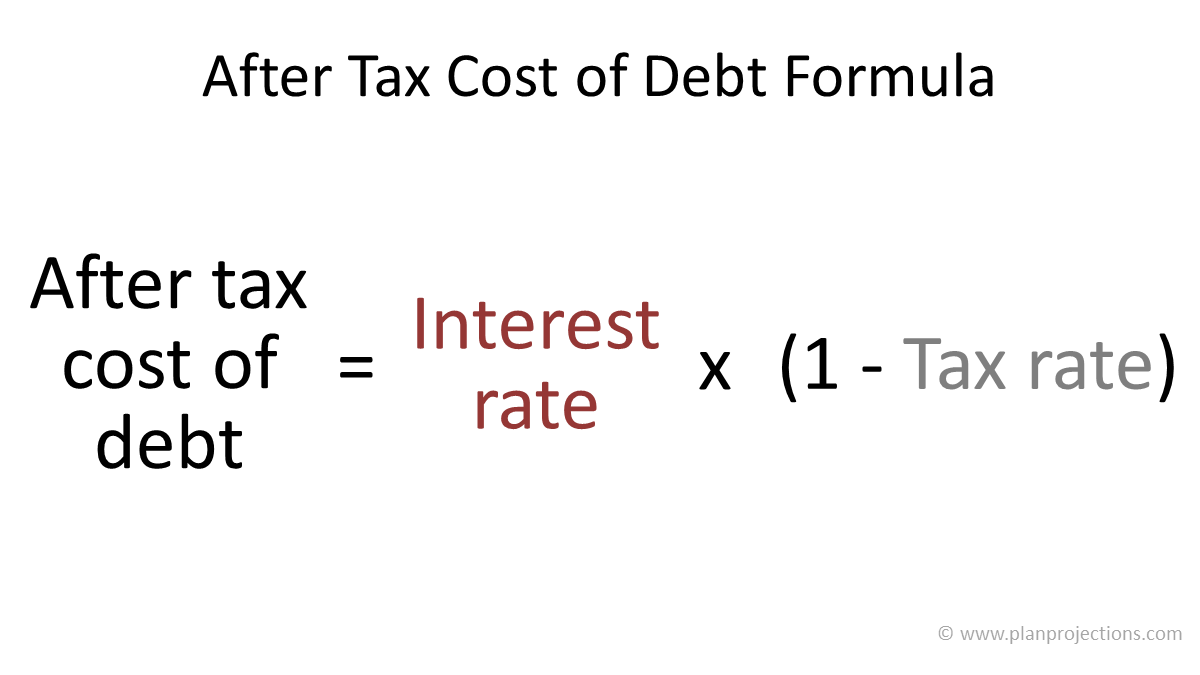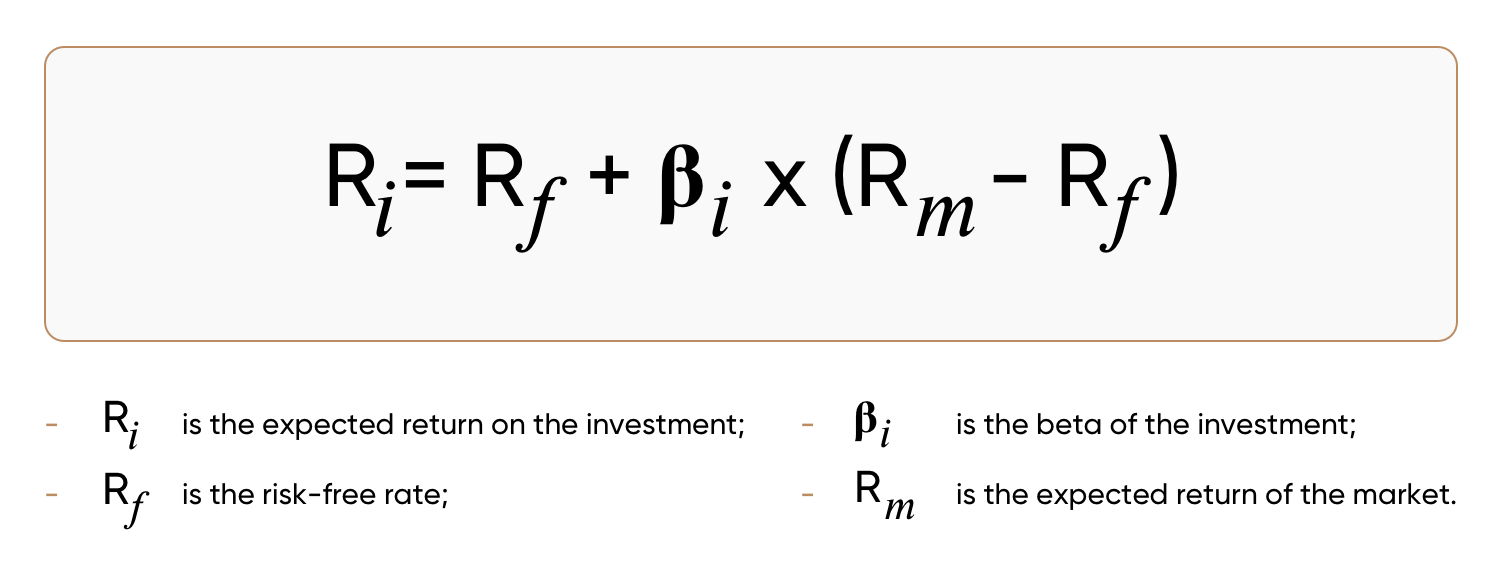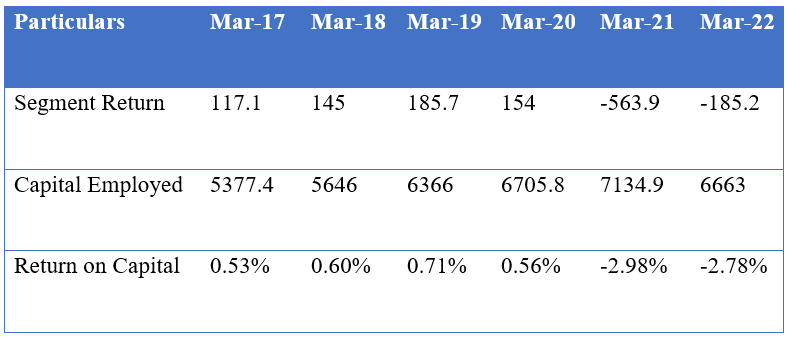What is the Cost of Capital? Why it is Important for Business and How to Calculate It?

# What is the Cost of Capital? Why it is Important for Business and How to Calculate It?

Published on 27 July 2022 .Views 46 .Comments 0
Share On

In this article, we will be discussing some important financial terms like Cost of Capital, Cost of Debt, and Cost of Equity. So. Let’s get started.

What is the Cost of Capital?

• Cost of Capital or Weight Average Cost of Capital (WACC) is the minimum return required by a company to justify taking up projects.
• It is composed of the cost of debt which is the after-tax cost of debt and the cost of equity. If the return on capital employed is higher than the cost of capital, only then making the investments into a project/vertical can be said to be justified.
• The cost of debt is fairly easy to calculate. The interest rate is the rate charged by lenders to lend money to the company. This interest rate is reduced by the tax shield on the interest.
• The formula for Calculating the Cost of Debt is:• The interest paid on debt is a tax-deductible expense. Tax shield in simple terms means the amount of tax that the company was required to pay lesser because of the interest expense. This is a notional inflow. Hence, the interest rate should be reduced by the tax shield on debt to arrive at the cost of debt
• Calculation of the cost of equity is not as simple. In the case of debt, the cost measurement can be based upon the interest charged by lenders. However, in the case of equity, the cost of equity can be subjective as the calculation depends on the expected return.
• The most popular method to calculate the cost of equity is CAPM (Capital Asset Pricing Model) which was Noble Prize-winning economist William Sharpe. Alternatively, the cost of equity is also calculated using dividend yield as a base. The cost of equity can be calculated as:Few Examples:

1. ITC Limited:

Numbers of ITC’s Hotel business division:• If the return on capital is higher than the cost of capital, the investment makes sense. The return on capital for the hotel division of the company has been consistently low. ITC has negligible debt on its balance sheet. Even after considering a conservative estimate of 10% cost of equity, the return on capital in this division of the company is low. This is where we can conclude the capital allocation in this vertical of the company going wrong.
1. Reliance Industries:
• After announcing aspirations of being net debt-free, Reliance Industries recently announced raising further debt to refinance. Taking on debt might not be desirable for certain investors as leverage brings risks. However, the cost of debt is much lower than the cost of equity.
• Hence, this could be good for a company like Reliance which has high operating cash flows to fund the debt repayment in future years.

Difference between Cost of Equity and Return on Equity:

• From the perspective of the business, the Cost of Equity is a cost, and Return on Equity is an income.
• Cost of Equity is based on expectation, hence, could differ from person to person, and Return on Equity is based on actual return, hence, it is a factual number
• A risky business will have a higher cost of equity, Since the return on equity is a lagging number, it has no relation to the risk.

What Should Individuals Do?

Cost of Capital is an important financial term that is often used by companies in their financial reports and conference calls. Further, the Cost of Capital is also an important factor when making financial decisions for the company, hence this concept also provides the investor the insight that how the company is raising funds, what modes they are opting for, is the selected method fruitful, and such others via evaluating the cost of capital concept. Therefore, an investor needs to have a brief look at cost of the capital structure while making investing decisions.

Disclaimer: The information here is provided for reference purposes only and should not be misconstrued as investment advice. Under no circumstances does this information represent are commendation to buy or sell stocks or MF.

Attachments:#### You like to know more. We like that!

Please subscribe Model Portfolio Plan to get access of all premium model portfolio articles Only at Rs. 9,999.00/Year.PremiumFree